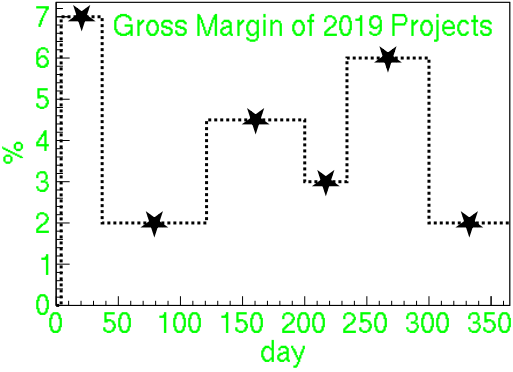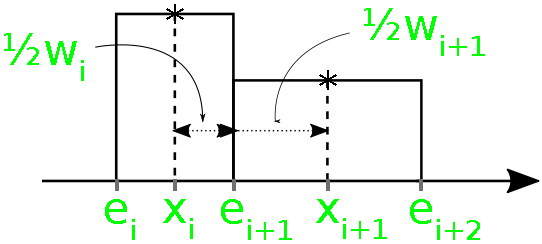# Histogram Reconstruction

• Last Updated : 17 Mar, 2021

In this article, we will discuss “Diagram to Histogram” also known as “Interval Finding”. While dealing with statistical data, diagrams are represented with single points(or the corresponding numbers) like the stars, which is supposed to be a histogram with a certain width as shown in the below figure.Analyzing the Problem:

In this problem statement, the assumption is that all intervals stick seamlessly together i.e., there are no gaps and no overlaps. The right edge of a bin is identical to the left edge of the following bin. Given N points, thus N bins, the task is to find (N + 1) bin edges. Every given point is located in the exact center of its X interval. This gives N equations for (N + 1) unknown quantities, so the system is underdetermined. There are two suggestions:

• The bins shall be as uniform. Mathematically, the variance of their bin widths shall be minimized.
• One supplies directly one fixed value for a bin edge, only the others are derived from that.

Calculations:

Here are few assumptions:

• Let xi be the coordinates of the point (their y values are completely irrelevant for us).
• wi the respective bin widths.
• ei the coordinates of the edges of the bin.
• Assume that the xi is sorted in ascending order.

Below is the image to illustrate the above concepts:From the above representation, two simple relations that can be easily verified are:The above formula simplifies the calculations especially for even N and is the reason why different results are obtained for odd and even values of N. The latter is directly the recursion formula that needs to fill in the array e[](the points on the X-axis of the histogram).

How to minimize the Variance?

The idea is similar to all the minimization problems i.e., check for the derivative 0. The problem is to reduce its formula to only one unknown variable that we can then work with it to find the minimum value.

• The variance is given by:• The value of mean value in the above formula is given by:• Derive the above equation with respect to an arbitrary quantity z as:• Applying iteratively the first equation derived above by replacing wi with (z = e0). For Example:• Putting all the above values obtained to find the value of e0:

When N is odd, thenWhen N is even, then• Alternatively, using the value of z = eN, gives a more simple formula as:

When N is odd, thenWhen N is even, thenApproach: The idea to solve the given problem is to iterate two nested loops, one for find the value of e0 or eN according to the derived formula and another loop for finding the elements of the array e[]. Therefore, the time complexity will be O(N) for all variants. Both loops work recursively and the second loop find the elements of the array e[] from the previous according to the second equation given above.

Note:

Below is the implementation of the above approach:

## C

 // C program for the above approach  #include  #include  #define N 6     // Function to fill the array elements  // e[] from the end  double* pointsToIntervalsN(      int n, const double* x,      double* e)  {      // Check for array overlap      if (n < 2 || !x || e < x && e + n >= x)          return NULL;         // If e is a NULL pointer, then      // allocate the array      if (e          || (e              = (double*)malloc(                  (n + 1) * sizeof(double)))) {             // Find the value of m on the          // basis of odd or even value of N          const int m = n & 1 ? n : 2;          const int j = m * n;          register double sum = 0.;             // Count i and x downwards          for (int i = m / 2; i < j; i += m) {              sum = i * *x++ - sum;          }          sum /= j / 2;             // Note: m/2 and j/2 above are          // integer divisions!          for (e[n] = sum; n--; e[n] = sum)              sum = 2 * *--x - sum;      }         // Including e==NULL for the case      // of malloc error      return e;  }     // Function to fill the output array  // from the front  double* pointsToIntervals0(const int n,                             const double* x,                             double* e)  {      // Check for overlaps      if (n < 2 || !x || e >= x && e < x + n)          return NULL;         if (e          || (e              = (double*)malloc(                  (n + 1) * sizeof(double)))) {             const int m = n & 1 ? n : 2;          const int j = m * n;          register double sum = 0.;             // Count i down and x          // from the front          x += n;             for (int i = m / 2; i < j;               i += m) {              sum = i * *--x - sum;          }             // Update the value of sum          sum /= j / 2;             *e = sum;          for (int i = 0; i < n;               e[++i] = sum)              sum = 2 * x[i] - sum;      }         // Return the updated e      return e;  }     // Function to find thefixed single  // e value from which all other e's  // are derived  double* pointsToIntervalsFix(const int n,                               const double* x,                               double e_base,                               double* e)  {      // Base Case      if (n < 1 || !x)          return NULL;         int k = 0;         // Perform Binary Search for e_base      for (int l = n; l > 1; l /= 2)          if (e_base > x[k + l / 2])              k += (l + 1) / 2;         // The e_base is either the left      // or the right edge of the bin      // around x[k]      if (e_base > x[k])          ++k;         // Now it's the left.         // Assume e is filled the left side      // first, the right side of e can      // overlap with x      if (e + k >= x && e < x + n)          return NULL;         // If the right side is filled      // first, so that the left side      // of e can overlap with x      if (e || (e = (double*)malloc(                    (n + 1) * sizeof(double)))) {          e[k] = e_base;             // Fill in both sides of array          // e[] starting from k          for (int i = k; i--; e[i] = e_base)              e_base = 2 * x[i] - e_base;             for (e_base = e[k]; k < n;               e[++k] = e_base)              e_base = 2 * x[k] - e_base;      }         return e;  }     // Driver Code  int main()  {      double e_orig[N + 1]          = { 4, 37, 121, 200, 234, 300, 365 };      double x[N], e_recN[N + 1], e_rec0[N + 1];      double e_base = 235.4, e_fix[N + 1];         // Make x the mean values of the      // neighbouring e_orig values:      for (int i = N; i--;           x[i] = (e_orig[i + 1] + e_orig[i]) / 2)          ;         // Function Call      pointsToIntervalsN(N, x, e_recN);      pointsToIntervals0(N, x, e_rec0);      pointsToIntervalsFix(N, x, e_base, e_fix);         printf("Example for n = %d:", N);      printf("\nx     ");      for (int i = 0; i < N; ++i)          printf("% .3f", x[i]);         printf("\ne_orig ");         for (int i = 0; i <= N; ++i)          printf("% .3f", e_orig[i]);         printf("\ne_recN ");         for (int i = 0; i <= N; ++i)          printf("% .3f", e_recN[i]);         printf("\ne_rec0 ");         for (int i = 0; i <= N; ++i)          printf("% .3f", e_rec0[i]);         printf("\ne_fix  ");         for (int i = 0; i <= N; ++i)          printf("% .3f", e_fix[i]);         return 0;  }

Output:

Example for n = 6:
x      20.500 79.000 160.500 217.000 267.000 332.500
e_orig  4.000 37.000 121.000 200.000 234.000 300.000 365.000
e_recN  3.583 37.417 120.583 200.417 233.583 300.417 364.583
e_rec0  3.583 37.417 120.583 200.417 233.583 300.417 364.583
e_fix   5.400 35.600 122.400 198.600 235.400 298.600 366.400


Caveats and Prospects:

• Both the methods i.e., the minimum variance and fixed edge occasionally fail in such a way that one or more histogram bins with negative width is obtained, i.e., there are some ei > e(i + 1) seemingly not properly sorted. This always happens when any random X values is taken as input.
• Try fixing the bin with the “most negative” width of all, hoping that all others will then look reasonable too.
• This brings us to the prospects because the two methods illustrated above are by far not the only possibilities to formulate an extra condition. Instead of “possibly equal” bin widths, assume a tendency like linear increase of the wi or an absolute minimum of all wi.

My Personal Notes arrow_drop_up
Recommended Articles
Page :